Heinz Spiess
EMME/2 Support Center, Haldenstrasse 16, CH-2558 Aegerten, Switzerland
heinz@spiess.ch

May 1990

Abstract:

In the past, many different models using observed link volumes to estimate or adjust O-D matrices have been proposed. While these models differ greatly in their mathematical formulation and interpretation, they all share the fact that using them for real size networks is difficult, if not impossible. This is due to the complexity of the computations that are involved and the need for very specialized software to carry them out. In this paper, we present a new gradient based model, which can and has been applied to large scale networks. Mathematically, the model is formulated as a convex minimization problem where, by following the direction of the steepest descent, we ensure that the original O-D matrix is not changed more than necessary. We show how this demand adjustment model can be implemented without the need to develop any new software, but only by using existing features of a standard transportation planning package (EMME/2). Since one iteration of the adjustment procedure consists essentially in two equilibrium assignments on the considered network, the method can be applied even to large scale networks and matrices. So far, our model has been applied successfully in several urban and national scale projects in Switzerland, Sweden and Finland, using networks with up to 522 traffic zones and 12460 links. Some results of these studies will be shown.

Introduction

In almost all transportation planning applications, the input data which is the most difficult and expensive to obtain is the origin-destination demand matrix. Since the demand data cannot be observed directly, it must be collected by carrying out elaborate and expensive surveys, involving home- or road-based interviews or complicated number plate tagging schemes. By contrast, observed link volumes can be obtained easily and with reasonable precision by simply counting the traffic at certain countpost links, either manually or automatically, using mechanical or inductive counting devices.

Thus, it is not surprising that a considerable amount of research has been carried out to investigate the possibility of estimating or improving an origin-destination demand matrix with observed volumes on the links of the considered network.

Many models have been proposed in the past, see e.g . Van Zuylen and Willumsen (1980), Van Vliet and Willumsen (1981), Nguyen (1982), Van Zuylen and Branston (1982) and Spiess (1987). These models, while being very interesting from a theoretical point of view, have been of relatively little practical relevance so far. This is due to immense computation time and storage requirements that arise in practical implementations and which limit these approaches to very small problem sizes only. As to our best knowledge, none of these methods has so far been successfully applied to large scale networks with hundreds of traffic zones and thousands of network links.

Most of these "traditional" approaches can be formulated as convex optimization problems in which the objective function corresponds to some distance function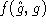between a "a-priori" demand matrix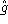and the resulting demand g. The constraints are then used to force the assigned volumes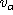to correspond to the observed volumes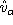on the count post links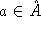. (Note that in some formulations, such as Van Zuylen and Branston (1982), these constraints are relaxed and, thus, appear as additional terms in the objective functions.)

In the following sections, we describe a new model which is suitable for large scale applications. We show how this model can be implemented without the need to develop any new programs, but by using the standard version of the transportation planning package EMME/2. Finally, we summarize the results of some urban and national scale applications in which the new model has been used recently.

In this paper a new type of model is proposed. It is also formulated as an optimization problem, but here the objective function to be minimized is a measure of distance between observed and assigned volumes. The simplest function of this type is the square sum of the differences, which leads to the following convex minimization problem: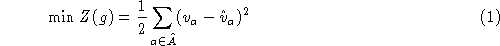subject to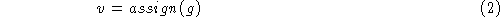where the ``pseudo''-function assign(g) is used to indicate the volumes resulting from an assignment of the demand matrix g. Of course, the particular assignment model used must correspond to a convex optimization problem, in order for (1) to be convex.

For the purpose of this paper, we shall assume that the term assignment refers to an equilibrium assignment, where a set of non-decreasing link cost functions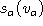on all links of the network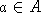ensures the convexity of the model. This type of equilibrium assignment problem has been extensively studied and can be solved efficiently, either by the successive linear approximation (Franck and Wolfe, 1956; Le Blanc et al., 1975; Florian, 1977) or the more recent PARTAN method (Florian et al, 1987; Arezki and Van Vliet, 1990).

Since the matrix estimation problem as formulated in (1) is highly underdetermined, it usually admits an infinite number of optimal solutions, i.e. possible demand matrices which all reflect the observed volumes equally well. Of course, in the actual planning context, we expect the resulting matrix to resemble as closely as possible the initial matrix, since it contains important structural information on the origin-destination movements. Therefore, just finding one solution to problem (1) is clearly not enough.

``Traditional'' models eliminate (at least partially) this problem of degeneracy by using an objective function Z(g) which corresponds to a measure of distanceand imposing the equality between observed and assigned values as constraints. While this approach indeed provides a mean to choose the ``best'' demand matrix (according to some criterion) among those satisfying the conditions on the observed volumes, it also increases significantly the complexity of the problem to be solved and, thus, contributes heavily to the fact that these models are so difficult to apply to large scale problems.

If we would have a solution algorithm which inherently finds a solution close to the starting point, we could leave the objective function (1) as is. Fortunately, the gradient method, also called the method of steepest descent, has exactly this property that we look for. It follows always the direction of the largest yield with respect to minimizing the objective function and, thus, it also assures us not to deviate from the starting solution more than necessary.

In the simplest case, when taking the gradient directly with respect to the variables g, the gradient method could be formulated as followswhere the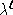have to be chosen small enough to ensure that the path followed by the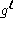is sufficiently near to the true gradient path. Note that we use the index i to denote an origin-destination pair (O-D pair), and that the set of all active O-D pairs is I.

However, if the gradient is based on the variables g as in (3), this implies that changes to the demand matrix are measured in an absolute way, i.e. number of trips, regardless of the relative change this would mean to the initial matrix. In particular, this would imply that O-D pairs with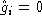would be affected by the adjustment as well.

For a more realistic approach the gradient should be based on the relative change of the demand, which can be written asNote that when the relative gradient is used, the algorithm becomes multiplicative in ghi. Therefore, a change in demand is proportional to the demand in the initial matrix and, in particular, zeros will be preserved by the process.

Before turning our attention to the evaluation of the gradient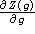, let us first look at the decomposition of the link volumesinto path flows. Let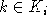denote the set of paths used for each O-D pair i, ieI, and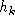the vector of the corresponding path flows. The link volumes can then be expressed as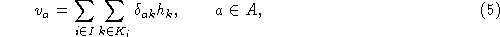where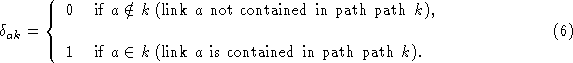Using the path probabilities instead of the path flows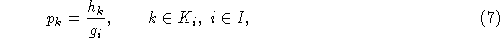equation (5) can be rewritten as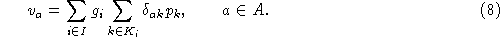We can now proceed to compute the gradient. Taking the derivative of (1), we obtain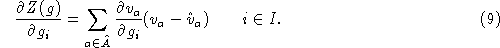Assuming that the path probabilities are locally constant, we obtain from (8)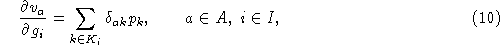which, when inserted into (9), yields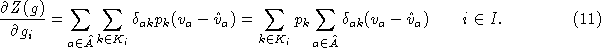In order to implement the gradient method 4, we also need to provide values for the step lengths. Choosing very small values for the step length has the advantage of following more precisely the gradient path, but has the disadvantage of requiring more steps. On the other hand, when choosing too large values for the step length, the objective function Z(g) can actually increase and the convergence of the algorithm would be lost. Thus, the optimal step length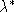at a given demand g can be found by solving the one-dimensional subproblem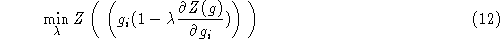subject to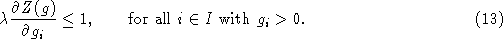Since the objective function Z is expressed in terms of the link volumes, we need to know how these change along the gradient direction. This can be obtained by applying the chain rule to (10)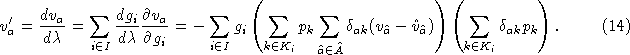Solving the minimization problem (12) can be done by finding the zero of the derivative. Applying again the chain rule we obtain the derivative as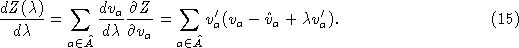This leads directly to the optimal step lengthwhich, in order to be feasible, needs only to be checked against and eventually be bounded by (13).

With the equations (11), (14) and (16), we have all the necessary results in order to solve the matrix adjustment problem (1) using the relative gradient method (4).

Implementation

One major practical difficulty for applying matrix estimation models in practice is due to the fact that most models can only be implemented in highly specialized computer programs which are difficult to obtain and operate, if they exist at all.

In this section we show that the gradient method can be implemented using the standard version of the widely used EMME/2 transportation planning software (Spiess 1984; INRO 1989). Since Release 3.0 of EMME/2 (released October 1987), a feature of the car equilibrium assignment module known as Additional Options Assignment is available. The aim of this feature is to provide a general framework for the simultaneous computation of various path dependent informations which might be needed in addition to the usual assignment results.

Let us briefly look at the mathematical interpretation of this feature of EMME/2. (The emphasized terms in the paragraphs below correspond to the nomenclature used in EMME/2.)

For each path generated during the normal equilibrium assignment, an additional path attribute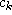is computed by combining the additional link attributes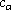of the link on the path with the path operator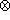(which can be any operator such as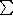,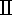,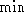or)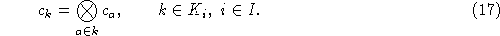Checking the path attributeagainst the specified path threshold interval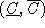determines if the path is included in the subsequent slave assignment of the additional demand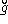. This way, the set of active paths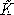is defined.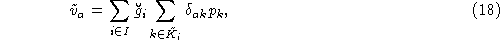and the additional attribute matrix, which can be one of the following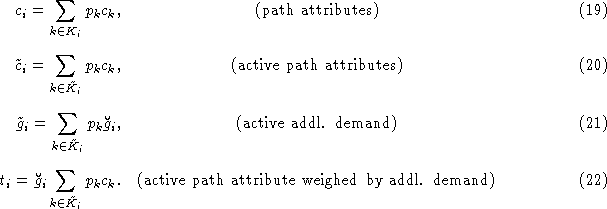Once we have expressed the additional options feature of EMME/2 in mathematical terms, it is evident that the framework does not only serve for the ``usual'' applications, such as link select analysis, partial assignments, or the computation of cost or distance matrices. It can also be used, as is, to implement the gradient method for the matrix adjustment problem, as described in the preceding section. The following paragraphs give an outline how this is achieved.

At the beginning of each iteration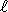of the gradient method, a ``plain'' equilibrium assignment (i.e. not using the additional options feature) is carried out with the current demand, in order to compute the link volumesi,. With these volumes, the additional link attributeis computed using the network calculator as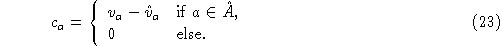The gradient matrixdefined in (11) is next computed with (22) as additional attribute matrix. This matrix is then used as additional demand matrixand the variables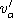of (14) are computed according to (18). The optimal step length (16) is evaluated again with the network calculator. Finally, the demand matrix is updated using the matrix calculator according to (4).

Using the macro feature of EMME/2, which allows the different modules of EMME/2 to be combined into more complex procedures, the entire algorithm can be packaged into one macro command, hiding all the implementational details from the user.

In terms of computation times, each iteration of the gradient method corresponds to one equilibrium assignment without additional options and two equilibrium assignments with additional options, plus some minor matrix and network calculations. While this is a substantial effort for every iteration, it is still doable even for the largest networks that can be handled in EMME/2, i.e. networks of up to 1600 traffic zones (2,560,000 O-D pairs!) and 32000 links.

Applications

During 1988 and 1989, we had the opportunity to test the proposed gradient method and apply it in practice in several studies. The test example used was based on the standard EMME/2 Winnipeg Demonstration Database. The applications include two urban applications for the cities of Bern and Basel and the two national road networks of Sweden and Finland. The table below gives some characteristics for the applications: number of traffic zones, number of network links, number of links with observed volumes, the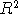-value between observed and assigned volumes using the initial demand, the-value after the adjustment, and, finally, the number of gradient iterations that were carried out for the adjustment.

 Study Zones Links Counts initialfinalIter. Winnipeg 154 2983 70 0.834 0.971 11 Basel 330 220 229 0.923 0.970 6 Bern 226 2676 338 0.960 0.995 20 Sweden 522 3879 334 0.827 0.992 20 Finland 469 12476 5759 0.491 0.886 30

As an example, the scattergrams of observed versus assigned volumes before and after the adjustment are shown in Figures 1 and 2 below for the Swedish national road network application. In Figure 3, the value of the objective function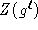is shown for all 20 iterations. The computations were carried out on a SUN SPARCstation 1 computer. On this equipment, each iteration of the gradient method took about 25 minutes of computing time.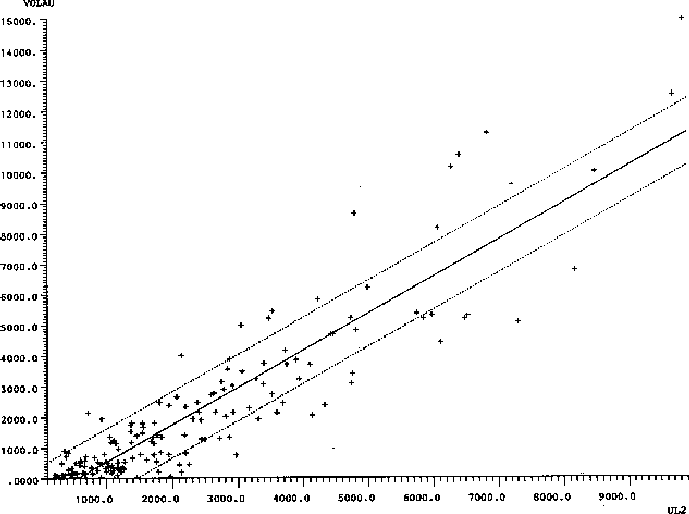Figure 1: Assigned vs observed volumes before adjustment.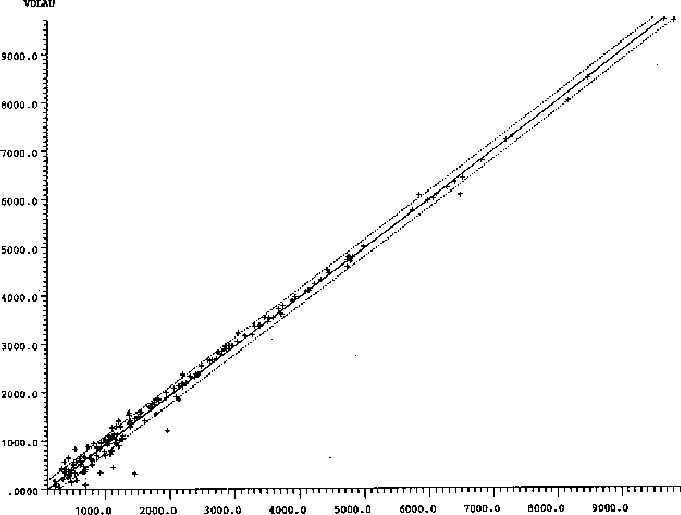Figure 2: Assigned vs observed volumes after adjustment.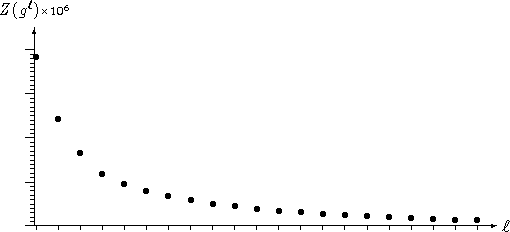Figure 3: Values of the objective function.

In all applications that were carried out so far, the gradient method was found to perform very well. However, there are some important practical points to consider before applying the algorithm:

• This model will adjust the demand matrix in order to better reflect the observed volumes. It should only be applied if all the other data which are used for the assignment have been extensively validated. The adjustment procedure will attempt to compensate any remaining error in the network coding, the volume delay functions or the observed volumes by modifying the demand matrix. This, of course, will not correct the error, but just add another error on top of it.
• The selection of the count post links is very important. Before applying the adjustment procedure, it is good practice to perform a rigorous count post analysis (this can also be carried out very elegantly using the additional options assignment feature of EMME/2). The count posts links should cover the network sufficiently, so that most trips will at least be counted once. Also the count post links should not carry a lot of local (= intrazonal) trips, since these will not be accounted for in the assignment. Finally, great care must be taken when the count post links are close to important connector links, since these are aggregated abstract trip ends which do not represent the true final destinations.
• Do not expect the observed volumes necessarily to form a consistent set. Inconsistencies can be due to inaccurate or non-simultaneous counts. But besides the imprecisions of the actual counting, inconsistencies can also arise from the zonal aggregation itself, which forces all trip ends to be at the centroid nodes. The counted volumes, however, are based on the true origin and destination of every trip. One should therefore not expect that the objective function Z must necessarily converge to zero.
• Once the adjustment has been carried out, it is crucial to analyze the results carefully and critically. We expect the change in the demand to be small and unsystematic. Large or systematic changes will almost always be an indication of a problem in the input data. Also, the comparison of the trip length histograms before and after the adjustment has proofed to be very valuable. In all applications so far the analysis of the first runs of the gradient method has lead to the discovery of network coding problems - even though some of the networks have been used for years.
• Every time corrections are made to the input data, the gradient method should be restarted with the initial demand matrix. Technically, it would be possible to make changes to the network or the observed volumes between iterations of the gradient method and then continue. But this would defeat the key property of the gradient method, which is to find a solution with minimal change to the initial demand.

Conclusions

We have formulated a new type of matrix adjustment model in which the steepest descent property of the gradient method is used to overcome the degeneracy problem. Since this method will inherently find a solution which is close to the starting point, it is not necessary to address explicitly the degeneracy of the solutions in the objective function. The simplicity of the proposed method makes it applicable even to large scale problems.

In this model, the path probabilities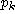need not to be stored explicitly, since the gradient matrixcan be built up directly as a by-product of the assignment. Therefore, the gradient model is not restricted to proportional assignments models, but is equally well applicable with the equilibrium assignment.

The method can be implemented without the need of any specialized software, but by just using the standard version of the EMME/2 transportation planning system. Therefore the gradient approach presented here is as much of theoretical interest as of practical importance, since it offers a simple analytic alternative to the still widely used practice of ``manual demand matrix calibration''.

Even though all applications so far were using equilibrium assignments on highway networks, the gradient approach which we have developed here is general enough to be applied to different assignment models. In particular, it could also be used in the context of transit networks. In this case, instead of the highway network equilibrium assignment, the transit assignment based on optimal strategies (see Spiess, 1984; Spiess and Florian, 1989) would be used. The mathematical formulation for the transit case, as well as some tests with real applications will be the topic of further research.

Finally, it is important to note that the objective function (1) used in this paper is only the simplest of many possible choices. The same gradient approach can equally well be used to solve problems with any other objective function of the form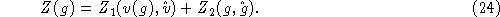Models of this type have been proposed by Willumsen (1984) and Brenninger-Göthe et al. (1989). The application of the gradient method in this more general context will be the topic of a forthcoming paper.

References

1
Arezki Y. and Van Vliet D. (1990). A full analytical implementation of the PARTAN/Frank-Wolfe algorithm for equilibrium assignment. Transport. Sci. 24, 58-62.

2
Brenninger-Göthe M., Jörnsten K.O. and Lundgren J.T. (1988). Estimation of origin-destination matrices from traffic counts using multi-objective programming formulations. Publication 88-14, Department of Mathematics, University of Linköping.

3
Florian M. (1977). An improved linear approximation algorithm for the network equilibrium (packet switching) problem. IEEE Proc. Decision and Control, 812-828.

4
Florian M., Guélat J. et Spiess H. (1987). An efficient implementation of the PARTAN variant of the linear approximation for the network equilibrium problem. Networks 17, 319-339.

5
Franck M. and Wolfe P. (1956). An algorithm for quadratic programming. Naval Res. Logist. Quart. 3, 95-110.

6
INRO Consultants Inc. (1989), EMME/2 User's Manual.

7
Le Blanc L.J., Helgason R.V. and Boyce D.E. (1985). Improved efficiency of the Franck-Wolfe algorithm for convex network problems. Transport. Sci. 19, 445-462.

8
Nguyen S. (1982). Estimating origin-destination matrices from observed volumes. Proceedings of the First Course on Transportation Planning Models of the International School of Transportation Planning, Amalfi, Italy, October 1982.

9
Spiess H. (1984). Contributions à la théorie et aux outils de planification de réseaux de transport urbain. Ph.D. thesis, Département d'informatique et de recherche opérationnelle, Centre de recherche sur les transports, Université de Montréal, Publication 382.

10
Spiess H. (1987). A maximum likelihood model for estimating origin-destination matrices. Transpn. Res. B 21, 395-412.

11
Spiess H. and Florian M. (1989). Optimal strategies: A new assignment model for transit networks Transpn. Res. B 23, 83-102.

12
Van Vliet D. and Willumsen L.G. (1981). Validation of the ME2 model for estimating trip matrices from traffic counts Proceedings of The Eights International Symposium on Transportation and Traffic Theory, June 1981.

13
Van Zuylen H.J. and Branston D.M. (1982). Consistent link flow estimation from counts. Transpn. Res. B 16, 473-476.

14
Van Zuylen H.J. and Willumsen L.G. (1980). The most likely trip matrix estimated from traffic counts. Transpn. Res. B 14, 281-293.

15
Willumsen L.G. (1984). Estimating time-dependent trip matrices from traffic counts. Ninth International Symposium on Transportation and Traffic Theory, VNU Science Press, 397-411.
...method
We shall use the term gradient throughout this paper. However, if the the link cost funtionsare not strictly increasing, there might be points at which the objective function Z(g) is not continuously differentiable. In this case, the occurrencies of the term gradient should be replaced by the term subgradient.

...Finland
These applications were carried out in collaboration with Rapp AG, Basel (Basel and Bern networks), Transek AB, Stockholm (Swedish national road network) and Finnmap OY, Helsinki (Finnish national road network).

Heinz Spiess, EMME/2 Support Center
Tue Sep 24 17:15:57 MET DST 1996